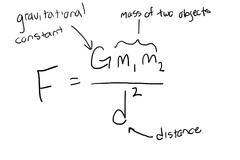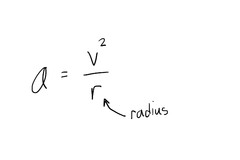College Physics Ch. 1-2Dennis Jennings
question

What are the fundamental physical quantities?

Distance, time, mass.
question

Distance

A measure of space in one dimension. Measured in meters.
question

Time

A process that repeats over and over at a regular rate. Measured in seconds.
question

Mass

A measure of how much matter is contained in it. Measured in grams.
question

Period

The time for one complete cycle of a process that repeats. Abbreviated as a capital T. Measured in units.
question

Formula for period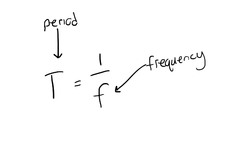question

Frequency

The number of cycles of a periodic process that occurs per unit time. Abbreviated as f. Measured in Hertz.
question

Formula for frequency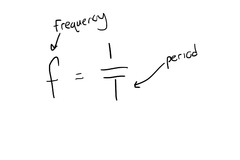question

What is an oscillation?

question

What is the frequency of a pendulum when the period is less than one second?

The frequency is always greater than 1 hertz.
question

Speed

Rate of motion. Rate of change of distance from a reference point. The distance something travels divided by the time elapsed. Measured in meters per second.
question

Formula for calculating average speed.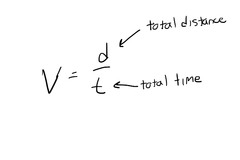question

Formula for calculating instantaneous speed.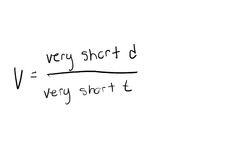question

Velocity

Speed in a particular direction.
question

What is the difference between speed and velocity?

Velocity is speed in a particular direction. Measured in meters per second.
question

Formula for velocity.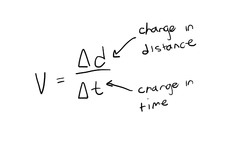question

Distance formula when speed is constant.

question

What is a vector?

A physical quantity that has both a numerical size (magnitude) and a direction associated with them.
question

What is a scalar?

A physical quantity having only magnitude, not direction.
question

The process process of adding two or more vectors together. The result of the vectors is called the resultant.
question

Acceleration

Rate of change of velocity. The change in velocity divided by the time elapsed.
question

Formula for acceleration.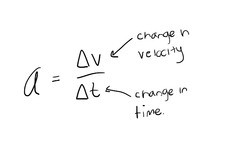question

3 types of acceleration.

Speed increase – linear Speed decrease – linear Direction change – centripetal
question

Centripetal acceleration.

The acceleration of an object in a circular direction. Centripetal means ‘center-seeking’
question

Formula for centripetal acceleration.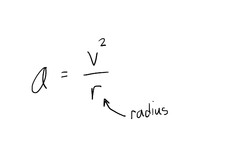question

What is the relationship between acceleration and velocity when something moves in a circle with constant speed?

It’s acceleration is perpendicular to its velocity.
question

What is zero velocity?

A situation in which no motion occurs.
question

Formula for velocity when acceleration is constant.question

What is ‘g’?

The acceleration due to the force of gravity. 9.80m/s^2. Abbreviated as ‘g’ Can be substituted for ‘a’ in acceleration equations.
question

Newton’s first law/Inertia

The tendency of an object in motion to remain in motion, or an object at rest to remain at rest, unless acted upon by a force.
question

Newton’s second law and formula

A force acting on a mass causes it to accelerate.
question

Weight/mass formula.

question

Newton’s third law

To every action there is always an equal and opposite reaction: or the forces of two bodies on each other are always equal and are directed in opposite directions.
question

Force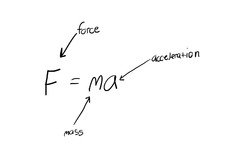A push or a pull acting on a body. Usually causes some distortion of the body, a change in its velocity, or both. Force is a vector. Measured is the newton (N).
question

How many newtons is one pound?

4.45 Newtons
question

How many pounds is one newton?

.225 lbs
question

Weight

The force of gravity acting on a body. Abbreviated W
question

Friction

A force of resistance to relative motion between two bodies or substances in physical contact.
question

Static friction

Friction when there is no relative motion between two objects.
question

For and object to move with constant velocity what has to happen?

The net force on it must be zero.
question

Centripetal force

Force directed toward the center of an objects path.
question

Newton

The force that would give a mass of one kilogram an acceleration of one meter per second per second.
question

Weight formula

question

Law of universal gravitation